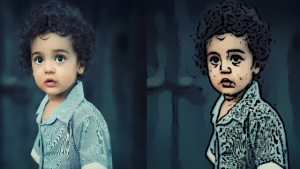Course Content
Introduction
OpenCV is an open-source computer vision library. OpenCV is an extremely optimized library with a focus on real-time applications.
0/1
Example
OpenCV is an open-source computer vision library. OpenCV is an extremely optimized library with a focus on real-time applications.
0/5
Private: OpenCV

In recent years, professional cartoonist software has risen all over the place but they’re rarely free. To achieve the basic cartoon effect, you don’t need great rendering software or even years of experience. Yes, that’s true!

All you need is essential – a bilateral filter and some edge detection. The bilateral filter will decrease the color palette, required for the cartoon look, and edge detection will enable the production of bold shapes.

• Implement the bilateral filter to decrease the color palette of the image.
• Transform the original color image to grayscale.
• Apply median blue to decrease image noise in the resultant grayscale image.
• Create an edge mask from the grayscale image applying adaptive thresholding.
• Integrate the color image from step 1 with the edge mask from step 4.

### Step 1

In this section, we are setting the parameters, reading the image, and resizing it.

```import cv2
import numpy as np

num_down = 2
num_bilateral = 7

print(img_rgb.shape)

img_rgb = cv2.resize(img_rgb, (500, 500))```

### Step 2

In this section, we are downsampling the image and then applying a bilateral filter, a specific amount of times

```img_color = img_rgb
for _ in range(num_down):
img_color = cv2.pyrDown(img_color)

for _ in range(num_bilateral):
img_color = cv2.bilateralFilter(img_color,
d=9,
sigmaColor = 9,
sigmaSpace = 7)```

### Step 3

In this section, we are upsampling, transforming the image to grayscale, applying the median blur, and the thresholding.

```for _ in range(num_down):
img_color = cv2.pyrUp(img_color)

img_gray = cv2.cvtColor(img_rgb,
cv2.COLOR_RGB2GRAY)
img_blur = cv2.medianBlur(img_gray, 7)

255,
cv2.THRESH_BINARY,
blockSize = 9,
C = 2)```

### Step 4

In this section, we perform ‘bitwise AND’ and then display the resultant image. Do make sure to add in the end: cv2.waitKey(0).

```img_edge = cv2.cvtColor(img_edge, cv2.COLOR_GRAY2RGB)
img_cartoon = cv2.bitwise_and(img_color, img_edge)

stack = np.hstack([img_rgb, img_cartoon])
cv2.imshow("Stack Images", stack)
cv2.waitKey(0)```

### Source code:

```import cv2
import numpy as np

num_down = 2
num_bilateral = 7

print(img_rgb.shape)

img_rgb = cv2.resize(img_rgb, (500, 500))

img_color = img_rgb
for _ in range(num_down):
img_color = cv2.pyrDown(img_color)

for _ in range(num_bilateral):
img_color = cv2.bilateralFilter(img_color,
d=9,
sigmaColor = 9,
sigmaSpace = 7)

for _ in range(num_down):
img_color = cv2.pyrUp(img_color)

img_gray = cv2.cvtColor(img_rgb,
cv2.COLOR_RGB2GRAY)
img_blur = cv2.medianBlur(img_gray, 7)

255,
cv2.THRESH_BINARY,
blockSize = 9,
C = 2)

img_edge = cv2.cvtColor(img_edge, cv2.COLOR_GRAY2RGB)
img_cartoon = cv2.bitwise_and(img_color, img_edge)

stack = np.hstack([img_rgb, img_cartoon])
cv2.imshow("Stack Images", stack)
cv2.waitKey(0)```

### The Output